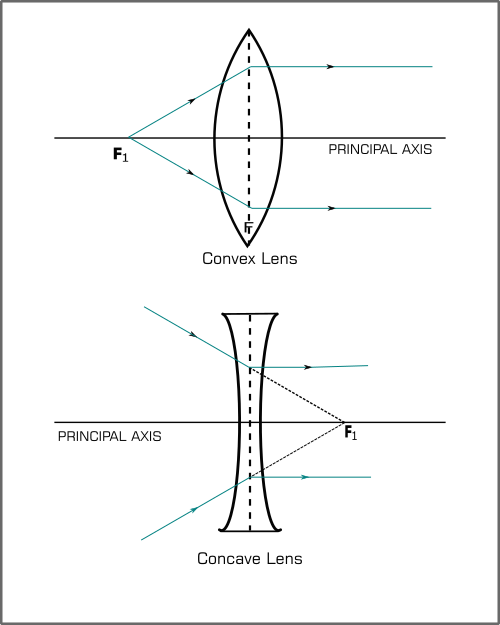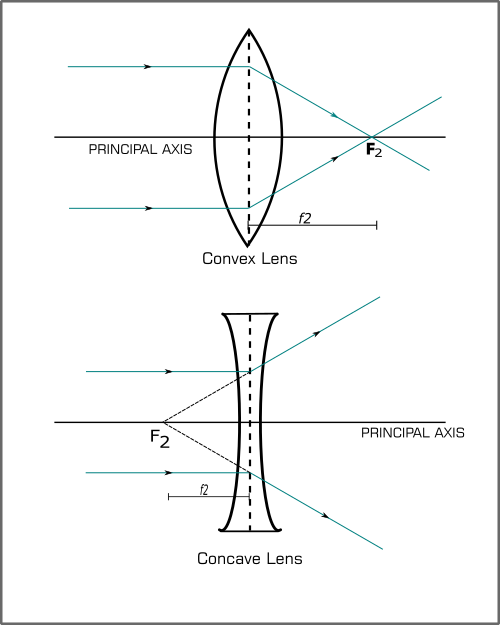FREE ASSISTANCE FOR THE INQUISITIVE PEOPLE
Tutorial Topics
Speed of Light and Reflective Index
Some Definition Related to a Lens - Physics Light
2905    Arnab De    18/02/2020### Some definition related to a lens

#### Centre of curvature:

A lens has two surfaces. Those two surfaces of the lens are the part of two different spheres. The centre of the sphere, whose part is the lens surface, is called the centre of the curvature of that surface of the lens. Every lens has two surfaces that mean each lens has two centre of curvature.

#### Principal axis:

It is a straight line which connects the two centre of curvature of a particular lens. In fig. C1C2 is the principal axis.#### Optical point:

Optical point is a point on the principal axis of the lens such that a ray of light passing through this point emerges parallel to its direction of incidence.

Optical centre of a thin lens is the point on the principal axis of the lens through which a ray of light passes undeviated.

In Fig. PQ is the incidence ray; it passes through QR in the lens. After refraction the ray pass through RS. Here PQ and RS are parallel. The ray intersects the principal axis at the point O. Thus here O is the optical point of the lens.

#### Principal foci:

A light ray can pass through a lens from either direction; therefore, a lens has two principal foci which are situated at equal distance from the optical centre, one on either side on the lens. These are known as the first focal point (or first focal) F1 and the second focal point (or second focus) F2.

##### First focal point
• For a convex lens, the first focal point is a point F1 on the principal axis of the lens such that the ray of the light coming from it, after refraction through the lens, becomes parallel to the principal axis of the lens.
• For a concave lens, the first focal point is a point F1 on the principal axis of the lens such that the incident rays of light appearing to meet at it, after refraction from the lens, becomes parallel to the principal axis of the lens.##### Second focal point
• For a convex lens, the second focal point is a point F2 on the principal axis of the lens such that the ray of the light incident parallel to the principal axis, after refraction from the lens, passes through it.
• For a concave lens, the second focal point is a point F2 on the principal axis of the lens such that the rays of the light incident parallel to the principal axis, after refraction from the lens, appear to be diverging from this point.#### First focal length

The distance from the optical centre O of the lens to its first focal point F1 is called the first focal length f1 of the lens.OF1 = f1.

#### Second focal length

The distance from the optical centre O of the lens to its first focal point F2 is called the second focal length f2 of the lens. OF2 = f2.

Speed of Light and Reflective Index
Author DetailsArnab De
I have over 16 years of experience working as an IT professional, ranging from teaching at my own institute to being a computer faculty at different leading institute across Kolkata. I also work as a web developer and designer, having worked for renowned companies and brand. Through tutorialathome, I wish to share my years of knowledge with the readers.
Enter New Comment
Comment History
No Comment Found Yet.By education, I mean all-around drawing of the best in child and man in body, mind and sprit.
Mahatma Gandhi
4297
40.91
Today So Far
Total View (Lakh)